# Designing Finite Automata from Regular Expression (Set 3)

• Last Updated : 20 Nov, 2019

In the below article, we shall see some Designing of Finite Automata form the given Regular Expression.

Regular Expression 1: ‘ab*’ (‘a’ followed by any number of ‘b’). The language of the given RE is,

`L1 = {a, ab, abb, abbb, .........} `

Its finite automata will be like below-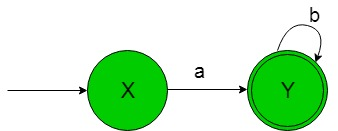In the above transition diagram, as we can see that state ‘X’ on getting ‘a’ as the input it transits to a final state ‘Y’ which on getting ‘b’ as the input it remains in the state of itself. Thus this FA accepting all the strings of the given RE language.

Regular Expression 2: ‘(ab)*’ (‘a’ followed by ‘b’ but substring ‘ab’ can be repeated any number of times). The language of the given RE is,

`L2 = {ε, ab, abab, ababab, .........} `

Its finite automata will be like below-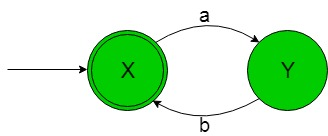In the above transition diagram, as we can see that initial and final state ‘X’ on getting ‘a’ as the input it transits to a final state ‘Y’ which on getting ‘b’ as the input it comes back to the state ‘X’. Thus this FA accepting all the strings of the given RE language.

Regular Expression 3: ‘(a+b)*’ (‘a’ union ‘b’ but substring ‘a+b’ can be repeated any number of times). The language of the given RE is,

`L3 = {ε, a, b, aa, aaab, bbbbb, ba, .......} `

The language containing ε and any number of ‘a’ or ‘b’ or both combined.
Its finite automata will be like below-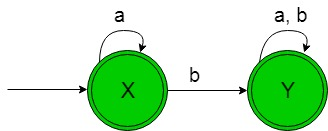In the above transition diagram, as we can see that state ‘X’ on getting ‘a’ as the input it remains in the state of itself and on getting ‘b’ as the input it transits to an another final state ‘Y’ which on getting ‘b’ or ‘a’ as the input it remains in the state of itself. Thus this FA accepting all the strings of the given RE language.

Note – The minimal DFA of regular expression (a+b)* will have only single state, which is both starting and final state. This will contain only loop of alphabet ‘a’ and ‘b’.

Regular Expression 4: ‘(ab+ba)*’ (‘ab’ union ‘ba’ but substring ‘ab+ba’ can be repeated any number of times). The language of the given RE is,

`L4 = {ε, ab, abab, abba, ba, baba, ........ } `

The language containing ε and any number of ‘ab’ or ‘ba’ or both combined.
Its finite automata will be like below-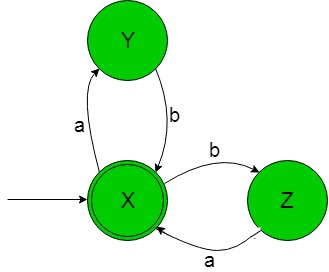In the above transition diagram, the initial and final state ‘X’ on getting ‘a’ as input it go to a state ‘Y’ and on getting ‘b’ as the input it go to an another state ‘Z’ and so on for the remaining states. Thus this FA accepting all the strings of the given RE language.

Regular Expression 5: ‘a+’ (Any number of ‘a’ excluding ε).
The language of the given RE is

`L5 = {a, aa, aaa, aaaa, .........} `

Its finite automata will be like below-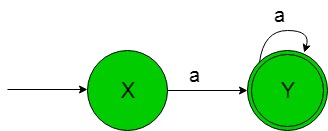In the above transition diagram, initial state ‘X’ on getting ‘a’ as the input it transits to a final state ‘Y’ which on getting ‘a’ as the input it remains in the state of itself. Thus this FA accepting all the strings of the given RE language.

My Personal Notes arrow_drop_up
Recommended Articles
Page :